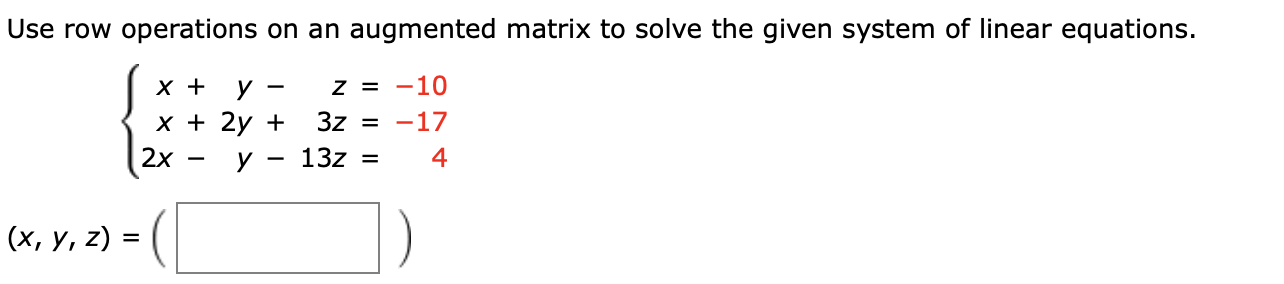# Use row operations on an augmented matrix to solve the given system of linear equations.х +z = -10yх + 2у +3z-172x13zy(х, у, 2) %3D

Question
1 viewshelp_outlineImage TranscriptioncloseUse row operations on an augmented matrix to solve the given system of linear equations. х + z = -10 y х + 2у + 3z -17 2x 13z y (х, у, 2) %3D fullscreen
check_circle

Step 1

According to the given information the given system of linear equation is:

Step 2

Now, put the system of equations into matrix form:

Step 3

Now apply the row transformation to convert the above matrix into augmented matrix.

A...

### Want to see the full answer?

See Solution

#### Want to see this answer and more?

Solutions are written by subject experts who are available 24/7. Questions are typically answered within 1 hour.*

See Solution
*Response times may vary by subject and question.
Tagged in

### Math